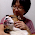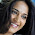# Overview

Fashion-MNIST is mnist-like image data set. Each data is 28x28 grayscale image associated with fashion. Literally, this is fashion version of mnist.

I'm thinking to use this data set on small experiment from now on. So, for the future, I checked what kind of data fashion-MNIST is.

On the article below, I explored from the viewpoint of Bayes.

#### Fashion-MNIST exploring using Keras and Edward

On the article, Fashion-MNIST exploring, I concisely explored Fashion-MNIST dataset. We can get access to the dataset from Keras and on this article, I'll try simple classification by Edward. I'll use Fashion-MNIST dataset. Fasion-MNIST is mnist like data set. You can think this as the fashion version of mnist.

# Fashion-MNIST

Fasion-MNIST is mnist like data set. You can think this as the fashion version of mnist. It is written that because mnist is too easy for classification and used too much, this data set was made. You can check the page to read the document of Fashion-MNIST.

Let’s see some images of the data set.

I randomly picked up some images. As you can see, this data set contains clothes, bags, pants and so on. The size of each image is 28x28 and those are gray-scaled. Literally, this is fashion version of mnist.

Just in case, let’s see the mnist data.

We can say that Fashion-MNIST is fashion version of mnist.

# Preparation

You can get the data from the page. This time, to use easy reader of the data, I just cloned the repository.

git clone https://github.com/zalandoresearch/fashion-mnist.git
cd fashion-mnist

# Classification

By some kinds of neural network model, I checked how difficult it is to classify, in a nutshell scale of accuracy.
At first, the code below reads data. As a premise, the code can be executed on the cloned directory to use easy reader of data.

%matplotlib inline
import random
import numpy as np
import matplotlib.pyplot as plt

fm_x_test, fm_y_test = mnist_reader.load_mnist('data/fashion', kind='t10k')

To see one of the images, we can plot.

plt.imshow(fm_x_train.reshape((28, 28)), cmap = 'gray')

## Simple model

The code below makes simple model for Fashion-mnist.

from keras.models import Model
from keras.layers import Dense, Input, Dropout
from keras.layers.normalization import BatchNormalization
from keras.utils import to_categorical
import keras
# simple model
def simple_model(x_train, y_train):
inputs = Input(shape=(784,))
x = BatchNormalization()(inputs)
x = Dense(128, activation='relu')(x)
x = Dense(256, activation='relu')(x)
x = Dense(128, activation='relu')(x)
predictions = Dense(10, activation='softmax')(x)
model = Model(input=inputs, output=predictions)
model.compile(loss=keras.losses.categorical_crossentropy,
metrics=['accuracy'])
history = model.fit(x_train, to_categorical(y_train), epochs=50, shuffle=True, validation_split=0.33)
return history

simple_history = simple_model(fm_x_train, fm_y_train)

To observe the training, prepare the plot function.

def show_history(history):
plt.plot(history.history['acc'])
plt.plot(history.history['val_acc'])
plt.ylabel('accuracy')
plt.xlabel('epoch')
plt.legend(['train_accuracy', 'test_accuracy'], loc='best')
plt.show()

The behavior of the training and the outcome of evaluation can be shown by the code below.

show_history(simple_history)
simple_history.model.evaluate(fm_x_test, to_categorical(fm_y_test))

The test accuracy by evaluation looks good but from the plot, we can see over-fitting.

[0.59005128074139357, 0.88780000000000003]

To ease over-fitting, I added some Dropout layers to the network. About Dropout, I wrote on this article, How Dropout works on Neural Network.

# simple model with dropout
def simple_model_dp(x_train, y_train):
inputs = Input(shape=(784,))
x = BatchNormalization()(inputs)
x = Dropout(0.2)(x)
x = Dense(128, activation='relu')(x)
x = Dropout(0.5)(x)
x = Dense(256, activation='relu')(x)
x = Dropout(0.5)(x)
x = Dense(128, activation='relu')(x)
x = Dropout(0.5)(x)
predictions = Dense(10, activation='softmax')(x)
model = Model(input=inputs, output=predictions)
model.compile(loss=keras.losses.categorical_crossentropy,
metrics=['accuracy'])
history = model.fit(x_train, to_categorical(y_train), epochs=50, shuffle=True, validation_split=0.33)
return history

simple_history_dp = simple_model_dp(fm_x_train, fm_y_train)
show_history(simple_history_dp)
simple_history_dp.model.evaluate(fm_x_test, to_categorical(fm_y_test))

The over-fitting itself was solved but there is no notable change.

[0.38736002795696256, 0.86939999999999995]

## Convolutional Neural Network

Next I made convolutional neural network model.

from keras.layers import Dense, Conv2D, MaxPooling2D, Flatten, Reshape

def conv_model(x_train, y_train):
inputs = Input(shape=(784,))
x = BatchNormalization()(inputs)
x = Dropout(0.2)(x)
x = Reshape((28, 28, 1))(x)
x = Conv2D(32, (3,3), activation='relu')(x)
x = Dropout(0.5)(x)
x = Conv2D(32, (3,3), activation='relu')(x)
x = Dropout(0.5)(x)
x = MaxPooling2D(pool_size=(2,2))(x)
x = Flatten()(x)
x = Dense(128, activation='relu')(x)
x = Dropout(0.5)(x)
predictions = Dense(10, activation='softmax')(x)
model = Model(input=inputs, output=predictions)
model.compile(loss=keras.losses.categorical_crossentropy,
metrics=['accuracy'])
history = model.fit(x_train, to_categorical(y_train), epochs=20, shuffle=True, validation_split=0.33)
return history

conv_history = conv_model(fm_x_train, fm_y_train)
show_history(conv_history)
conv_history.model.evaluate(fm_x_test, to_categorical(fm_y_test))

The training step and the evaluation are like this.

[0.33879369566440581, 0.88539999999999996]

From the viewpoint of accuracy scale, the model's accuracy tends to gather around 0.9, to say precisely 0.88.

## Related Article

#### Fashion-MNIST exploring using Keras and Edward

On the article, Fashion-MNIST exploring, I concisely explored Fashion-MNIST dataset. We can get access to the dataset from Keras and on this article, I'll try simple classification by Edward. I'll use Fashion-MNIST dataset. Fasion-MNIST is mnist like data set. You can think this as the fashion version of mnist.

1.This comment has been removed by the author.

2.This comment has been removed by the author.

3.Hey - thanks for writing this article. Very helpful to understand (especially dropouts). Can you also write something about regularisation.

Pls share how I can save these model weights? I intend to convert them into a .pb and retrain on a different classes of clothes.

Thanks

1.Thanks for the comment.
I'll write the articles about regularization and how to save the model, although actually because I just got married and bit busy, it takes bit time haha.

4.I like your blog, I read this blog please update more content on python, further check it once at python online training